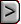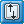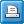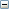﻿ HFB6 - Horizontal Flow Barrier Package
Online Guide to MODFLOW

HFB6 - Horizontal Flow Barrier PackageHFB6 - Horizontal Flow Barrier PackageHFB6 - Horizontal Flow Barrier PackageAbbreviation in Name file

HFB6

Purpose

The Horizontal Flow Barrier package is used to simulate barriers to flow such as slurry walls by reducing the conductance between individual pairs of cells.

Documentation

 • Harbaugh, A.W., Banta, E.R., Hill, M.C., and McDonald, M.G., 2000, MODFLOW-2000, the U.S. Geological Survey modular ground-water model -- User guide to modularization concepts and the Ground-Water Flow Process: U.S. Geological Survey Open-File Report 00-92, 121 p.
 • Harbaugh, A.W., 2005, MODFLOW-2005, the U.S. Geological Survey modular ground-water model -- the Ground-Water Flow Process: U.S. Geological Survey Techniques and Methods 6-A16. https://pubs.usgs.gov/tm/2005/tm6A16/
 • Harbaugh, A.W., 2005, MODFLOW-2005, the U.S. Geological Survey modular ground-water model -- the Ground-Water Flow Process: U.S. Geological Survey Techniques and Methods 6-A16. https://pubs.usgs.gov/tm/2005/tm6A16/
 • Hsieh, P.A., and Freckleton, J.R., 1993, Documentation of a computer program to simulate horizontal-flow barriers using the U.S. Geological Survey modular three- dimensional finite-difference ground-water flow model: U.S. Geological Survey Open-File Report 92-477, 32 p.
 • release.txt
 • ofr-00-92-errata.pdf
 • Hanson, R.T., Boyce, S.E., Schmid, Wolfgang, Hughes, J.D., Mehl, S.M., Leake, S.A., Maddock, Thomas, III, and Niswonger, R.G., 2014, One-Water Hydrologic Flow Model (MODFLOW-OWHM): U.S. Geological Survey Techniques and Methods 6–A51, 120 p., https://dx.doi.org/10.3133/tm6A51.

Related Packages

One of the flow packages, Block-Centered Flow package (BCF), Layer-Property Flow package (LPF) or the Hydrogeologic-Unit Flow package (HUF2) sets the conductance between cells that is modified by the Horizontal Flow Barrier package.

Supported in

 • MODFLOW-2000
 • MODFLOW-2005
 • MODFLOW-LGR
 • MODFLOW-CFP
 • MODFLOW-NWT
 • MODFLOW-OWHM

Other Notes

 • The "Model-Layer Variable-Direction Horizontal Anisotropy" (LVDA) capability is incompatible with the Horizontal Flow Barrier package.
 • The capability of routing flow between non-adjacent cells can only be used with the NWT solver and is only included in MODFLOW-OWHM.
 • For vertical barriers, the layers must be adjacent to one another.

Input Instructions

The Horizontal Flow Barrier Package is documented in Hsieh and Freckleton (1993). Input for the Horizontal Flow Barrier (HFB) Package is read from the file that has file type “HFB6” in the name file. The file type has been changed from that used in MODFLOW-96, which was “HFB”, because the input data are not compatible. All variables are read in free format.

FOR EACH SIMULATION

Data Set 0

[#Text]

Item 0 is optional—“#” must be in column 1. Item 0 can be repeated multiple times.Text
 Text—is a character variable (199 characters) that starts in column 2. Any characters can be included in Text. The “#” character must be in column 1. Except for the name file, lines beginning with # are restricted to these first lines of the file. Text is printed when the file is read.

Data Set 1

NPHFB MXFB NHFBNP  [MXHFBNP] OptionExplanation of variablesNPHFB
 NPHFB—is the number of horizontal-flow barrier parameters to be defined in Items 2 and 3. Note that for an HFB parameter to have an effect in the simulation, it must be defined in Items 2 and 3 and made active using Item 6 and 9 to have an effect in the simulation. In MODFLOW-2000, the maximum number of allowed parameters is 999.  In MODFLOW-2005,-LGR, and -CFP the maximum number of allowed parametersis 2000. In MODFLOW-OWHM, the maximum number of allowed parameters is specified in the Basic package.MXFB
 MXFB—is the maximum number of HFB barriers that will be defined using parameters.NHFBNP
 NHFBNP—is the number of HFB barriers not defined by parameters for current stress period. Item 4 will be read NHFBNP times as specified in item 1. If NHFBNP is set to -1 in item 7, then NACTHFB and items 8 and 9 are not read, and the barriers defined in the previous stress period are used. Item 8 will be read NHFBNP times as specified in item 7.MXHFBNP
 the maximum number of HFB barriers not defined by parameters used during any stress period. If MXHFBNP is not specified, it is set equal to NHFBNP in item 1. MXHFBNP is only included in MODFLOW-OWHM.Option

Option—is an optional list of character values.

 • NOPRINT—suppresses the printing of cell lists.

Repeat Items 2 and 3 for each NPHFB parameter. Items 2 and 3 are not read if NPHFB is negative or zero.

Data Set 2

PARNAM PARTYP Parval NLSTExplanation of variablesPARNAM
 PARNAM—is the name of a parameter. This name can consist of 1 to 10 characters and is not case sensitive. That is, any combination of the same characters with different case will be equivalent. All parameter names must be unique.PARTYP
 PARTYP—is the type of parameter. For the HFB Package, the only allowed parameter type is HFB, which defines values of the hydraulic characteristic of the barrier.Parval
 Parval—is the parameter value. This parameter value may be overridden by a value in the PVAL file, the Sensitivity Process (SEN) input file, or by a value generated by the Parameter-Estimation (PES) Process.NLST
 NLST—is the number of horizontal-flow barrier cells included in the parameter.

Data Set 3

Layer IROW1 ICOL1 IROW2  ICOL2 Factor [Layer2]

NLST repetitions of Item 3 are required; they are read by module ULSTRD. (SFAC of the ULSTRD utility module applies to Factor).Explanation of variablesLayer
 Layer—is the number of the model layer in which the horizontal-flow barrier is located.IROW1
 IROW1—is the row number of the cell on one side of the horizontal-flow barrier.ICOL1
 ICOL1—is the column number of the cell on one side of the horizontal-flow barrier.IROW2
 IROW2—is the row number of the cell on the other side of the horizontal-flow barrier.ICOL2
 ICOL2—is the column number of the cell on the other side of the horizontal-flow barrier.Factor
 Factor—is the factor used to calculate hydraulic characteristic from the parameter value. The hydraulic characteristic is the product of Factor and the parameter value. If final hydraulic characteristic is negative, then it acts as a multiplier to the conductance between the two model cells specified as containing a barrier. For example, if the final result was –1.5, it would multiply by 1.5 the conductance between the two cells. Negative Factor values are only supported in MODFLOW-OWHM.Layer2
 the model layer of the second cell which contains a barrier. This cell is indexed as [IROW2, ICOL2, Layer2]. This variable is optional and if not present is set equal to Layer. If it is equal to Layer, then no flow routing occurs, and the barrier functions identically to previous versions of HFB. To use the flow routing capability, the NWT solver must be used. Layer2 is only included in MODFLOW-OWHM. If Layer2 is not equal to Layer, either IROW1 must equal IROW2 or ICOL1 must equal ICOL2. Typically either the absolute value of IROW1 - IROW2 equals 1 or the absolute value of ICOL1 - ICOL2 equals 1 For vertical barriers (IROW1 equals IROW2 and ICOL1 equals ICOL2), Layer and Layer2 must specify adjacent layers.

If IROW1=IROW2 and ICOL1=ICOL2 and ABS(Layer2- Layer)=1, then there is no flow routing, but instead the barrier is changed to a vertical barrier between layers and will work with any solver package.

FOR FIRST STRESS PERIOD OR ALL STRESS PERIODS

Data Set 4

Layer IROW1 ICOL1 IROW2  ICOL2 Hydchr [Layer2]

NHFBNP repetitions of Item 4 are read. Item 4 is not read if NHFBNP is negative or zero.Explanation of variablesLayer
 Layer—is the number of the model layer in which the horizontal-flow barrier is located.IROW1
 IROW1—is the row number of the cell on one side of the horizontal-flow barrier.ICOL1
 ICOL1—is the column number of the cell on one side of the horizontal-flow barrier.IROW2
 IROW2—is the row number of the cell on the other side of the horizontal-flow barrier.ICOL2
 ICOL2—is the column number of the cell on the other side of the horizontal-flow barrier.Hydchr
 Hydchr—is the hydraulic characteristic of the horizontal-flow barrier. The hydraulic characteristic is the barrier hydraulic conductivity divided by the width of the horizontal-flow barrier. If hydraulic characteristic is negative, then it acts as a multiplier to the conductance between the two model cells specified as containing a barrier. For example, if the final result was –1.5, it would multiply by 1.5 the conductance between the two cells.  Negative Hydchr values are only supported in MODFLOW-OWHM.Layer2
 the model layer of the second cell which contains a barrier. This cell is indexed as [IROW2, ICOL2, Layer2]. This variable is optional and if not present is set equal to Layer. If it is equal to Layer, then no flow routing occurs, and the barrier functions identically to previous versions of HFB. To use the flow routing capability, the NWT solver must be used. Layer2 is only included in MODFLOW-OWHM. If Layer2 is not equal to Layer, either IROW1 must equal IROW2 or ICOL1 must equal ICOL2. Typically either the absolute value of IROW1 - IROW2 equals 1 or the absolute value of ICOL1 - ICOL2 equals 1 For vertical barriers (IROW1 equals IROW2 and ICOL1 equals ICOL2), Layer and Layer2 must specify adjacent layers.

If IROW1=IROW2 and ICOL1=ICOL2 and ABS(Layer2- Layer)=1, then there is no flow routing, but instead the barrier is changed to a vertical barrier between layers and will work with any solver package.

Data Set 5NACTHFB
 NACTHFB—is the number of active HFB parameters.

Data Set 6

Pname

NACTHFB repetitions of Item 6 are read. Item 6 is not read if NACTHFB is negative or zero.Pname
 Pname—is the name of a parameter to be used in the simulation. NACTHFB parameter names will be read.

OPTIONAL: FOR EACH SUBSEQUENT STRESS PERIOD Data Sets 7-9 are only included in MODFLOW-OWHM

Data Set 7 (only in MODFLOW-OWHM)

NACTHFB NHFBNP

If NACTHFB is set to –1, then NHFBNP and items 8 and 9 are not read. Instead the barriers defined in the previous stress period are used. If MXHFBP is 0, then NHFBNP is not read in since it cannot be larger than 0.Explanation of variablesNACTHFB
 the number of active HFB parameters for the current stress period.NHFBNP
 the number of HFB barriers not defined by parameters for current stress period. Item 4 will be read NHFBNP times as specified in item 1. If NHFBNP is set to -1 in item 7, then NACTHFB and items 8 and 9 are not read, and the barriers defined in the previous stress period are used. Item 8 will be read NHFBNP times as specified in item 7.

Data Set 8 (only in MODFLOW-OWHM)

Pname

NACTHFB, from item 7, repetitions of Item 8 are read. Item 8 is not read if NACTHFB is negative or zero.Pname
 the name of a parameter to be used in the current stress period. NACTHFB parameter names will be read.

Data Set 9 (only in MODFLOW-OWHM)

Layer IROW1 ICOL1 IROW2 ICOL2 Hydchr [Layer2]

NHFBNP, from item 7, repetitions of Item 9 are read. Item 9 is not read if NHFBNP or MXHFBP is negative or zero.Explanation of variablesLayer
 Layer—is the number of the model layer in which the horizontal-flow barrier is located.IROW1
 IROW1—is the row number of the cell on one side of the horizontal-flow barrier.ICOL1
 ICOL1—is the column number of the cell on one side of the horizontal-flow barrier.IROW2
 IROW2—is the row number of the cell on the other side of the horizontal-flow barrier.ICOL2
 ICOL2—is the column number of the cell on the other side of the horizontal-flow barrier.Hydchr
 Hydchr—is the hydraulic characteristic of the horizontal-flow barrier. The hydraulic characteristic is the barrier hydraulic conductivity divided by the width of the horizontal-flow barrier. If hydraulic characteristic is negative, then it acts as a mulitplier to the conductance between the two model cells specified as containing a barrier. For example, if the final result was –1.5, it would multiply by 1.5 the conductance between the two cells.Layer2
 the model layer of the second cell which contains a barrier. This cell is indexed as [IROW2, ICOL2, Layer2]. This variable is optional and if not present is set equal to Layer. If it is equal to Layer, then no flow routing occurs, and the barrier functions identically to previous versions of HFB. To use the flow routing capability, the NWT solver must be used. Layer2 is only included in MODFLOW-OWHM. If Layer2 is not equal to Layer, either IROW1 must equal IROW2 or ICOL1 must equal ICOL2. Typically either the absolute value of IROW1 - IROW2 equals 1 or the absolute value of ICOL1 - ICOL2 equals 1 For vertical barriers (IROW1 equals IROW2 and ICOL1 equals ICOL2), Layer and Layer2 must specify adjacent layers.

If IROW1=IROW2 and ICOL1=ICOL2 and ABS(Layer2- Layer)=1, then there is no flow routing, but instead the barrier is changed to a vertical barrier between layers and will work with any solver package.